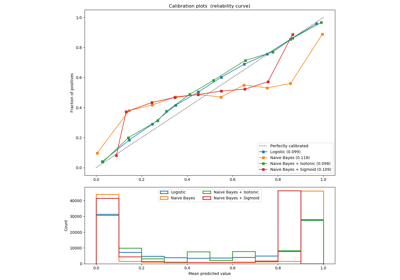/scikit-learn

# sklearn.metrics.precision_score

`sklearn.metrics.precision_score(y_true, y_pred, labels=None, pos_label=1, average=’binary’, sample_weight=None)` [source]

Compute the precision

The precision is the ratio `tp / (tp + fp)` where `tp` is the number of true positives and `fp` the number of false positives. The precision is intuitively the ability of the classifier not to label as positive a sample that is negative.

The best value is 1 and the worst value is 0.

Read more in the User Guide.

Parameters: `y_true : 1d array-like, or label indicator array / sparse matrix` Ground truth (correct) target values. `y_pred : 1d array-like, or label indicator array / sparse matrix` Estimated targets as returned by a classifier. `labels : list, optional` The set of labels to include when `average != 'binary'`, and their order if `average is None`. Labels present in the data can be excluded, for example to calculate a multiclass average ignoring a majority negative class, while labels not present in the data will result in 0 components in a macro average. For multilabel targets, labels are column indices. By default, all labels in `y_true` and `y_pred` are used in sorted order. Changed in version 0.17: parameter labels improved for multiclass problem. `pos_label : str or int, 1 by default` The class to report if `average='binary'` and the data is binary. If the data are multiclass or multilabel, this will be ignored; setting `labels=[pos_label]` and `average != 'binary'` will report scores for that label only. `average : string, [None, ‘binary’ (default), ‘micro’, ‘macro’, ‘samples’, ‘weighted’]` This parameter is required for multiclass/multilabel targets. If `None`, the scores for each class are returned. Otherwise, this determines the type of averaging performed on the data: `'binary':` Only report results for the class specified by `pos_label`. This is applicable only if targets (`y_{true,pred}`) are binary. `'micro':` Calculate metrics globally by counting the total true positives, false negatives and false positives. `'macro':` Calculate metrics for each label, and find their unweighted mean. This does not take label imbalance into account. `'weighted':` Calculate metrics for each label, and find their average weighted by support (the number of true instances for each label). This alters ‘macro’ to account for label imbalance; it can result in an F-score that is not between precision and recall. `'samples':` Calculate metrics for each instance, and find their average (only meaningful for multilabel classification where this differs from `accuracy_score`). `sample_weight : array-like of shape = [n_samples], optional` Sample weights. `precision : float (if average is not None) or array of float, shape = [n_unique_labels]` Precision of the positive class in binary classification or weighted average of the precision of each class for the multiclass task.

#### Examples

```>>> from sklearn.metrics import precision_score
>>> y_true = [0, 1, 2, 0, 1, 2]
>>> y_pred = [0, 2, 1, 0, 0, 1]
>>> precision_score(y_true, y_pred, average='macro')
0.22...
>>> precision_score(y_true, y_pred, average='micro')
0.33...
>>> precision_score(y_true, y_pred, average='weighted')
...
0.22...
>>> precision_score(y_true, y_pred, average=None)
array([0.66..., 0.        , 0.        ])
```

## Examples using `sklearn.metrics.precision_score`Probability Calibration curves